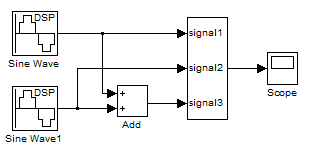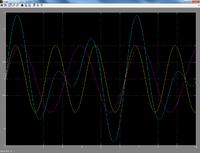# Find period of this signal

Status
Not open for further replies.

#### JupiW

##### Newbie level 1x(t) = Sin(2t) + Sin(3t)

Thnx#### prestonee

##### Full Member level 36t, it is 1st common number between them...just as sin(9t)+sin(3t) would have a period of 9t because its a common multiple of both.
this should be in homework section though, would request a move

#### Mityan

##### Full Member level 5Sin(a) + Sin(b) = 2*( sin((a+b)/2)*cos((a-b)/2) ). So this = 2*sin(2.5t)*cos(0.5t)

I think the period will be 2.5t. Am I right?

This is one of basic trigonomery equations. They are available in google and should be searched prior to asking there.

#### permuteIf the example were sin(2t)+sin(0.5t), then you would have a period of 4pi (seconds). likewise sin(2t) + sin(6t) would be periodic with pi (seconds). sin(6t) + sin(10t) would have a period of pi (seconds) as well. Using these examples, it should be easy to find the answer to what appears to be a homework question.

For sin(w*t), w would be an angular frequency (rad/second), not a period.

#### Mityan

##### Full Member level 5If the example were sin(2t)+sin(0.5t), then you would have a period of 4pi (seconds). likewise sin(2t) + sin(6t) would be periodic with pi (seconds). sin(6t) + sin(10t) would have a period of pi (seconds) as well. Using these examples, it should be easy to find the answer to what appears to be a homework question.

For sin(w*t), w would be an angular frequency (rad/second), not a period.
Indeed. In my example 2*pi*f = 2.5, so period T = 2*pi/2.5

#### albbgThe period of the sum of periodic signal is given by the least common multiple (lcm) among the periods of each signal.

In your example: x(t) = Sin(2t) + Sin(3t)

sin (and cos) function complete a period every 2Π rad, then (supposing to start from 0 rad):

2•t1=2Π --> t1=Π
3•t2=2Π --> t2=2•Π/3

to find lcm you have to ignore the denominator if it is an integer and take the factor having maximum exponent at the two numerators. In this case we have the factors Π (numerator of t1), 2 and Π (numerator of t2) so I'll take Π only once and 2.
lcm=2•Π ==> the period of x(t) will be 2•Π

As another example, let's consider x(t) = sin(1.3t)+sin(3t)

1.3•t1=2Π --> t1=2Π/1.3 --> I have to transform the denominator into integer --> t1=20Π/13
3•t2=2Π --> t2=2Π/3

The lcm (an then the period) will be now 20Π

factors were 2²,5,Π and 2,Π so I'll take 2²,5,Π

•Mityan

### Mityan

points: 2

#### Mityan

##### Full Member level 5The period of the sum of periodic signal is given by the least common multiple (lcm) among the periods of each signal.

In your example: x(t) = Sin(2t) + Sin(3t)

sin (and cos) function complete a period every 2Π rad, then (supposing to start from 0 rad):

2•t1=2Π --> t1=Π
3•t2=2Π --> t2=2•Π/3

to find lcm you have to ignore the denominator if it is an integer and take the factor having maximum exponent at the two numerators. In this case we have the factors Π (numerator of t1), 2 and Π (numerator of t2) so I'll take Π only once and 2.
lcm=2•Π ==> the period of x(t) will be 2•Π

As another example, let's consider x(t) = sin(1.3t)+sin(3t)

1.3•t1=2Π --> t1=2Π/1.3 --> I have to transform the denominator into integer --> t1=20Π/13
3•t2=2Π --> t2=2Π/3

The lcm (an then the period) will be now 20Π

factors were 2²,5,Π and 2,Π so I'll take 2²,5,Π
You are right.

Lets make a small simulink model:The frequencies of sources are 3/2/pi and 2/2/pi, cause 3 = 2*pi*f1, 2 = 2*pi*f2
The result diagram will be the following:As we can see from picture, the period of sum is 2*pi = 6.xxx

Status
Not open for further replies.